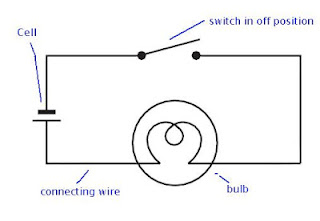## Electricity

### Worksheet

Fill in the blanks

1. The source of all electricity is ______. It’s SI unit is _________.

2. The net charge flowing through a cross section of a conductor in unit time is called ________.

3. Electric potential difference between points in an electric circuit is the ______ _______ to move a unit charge from one point to other. i.e. 1V = ____/1C.

4. Current is measured in Amperes using _______.

5. If 90 coulombs charge passes through the conductor for 5 minutes, then the current in the conductor is _____.

6. A _________ is an electronic measuring instrument the combines several measuring functions (electric potential difference, electric current and electric resistance) in one unit.

7. SI unit of electric potential difference is _______.

8. When the cells of 1V , 1.5V, 2V, are connected in series then the resultant potential difference is _____.

9. When resistances (R1, R2, R3...) are connected in series, their equivalent resistance is equal to the  of the individual resistances. i.e. R = __________

10. SI unit electric resistance is ________.

11. The resistance of a conductor is directly proportional to its _____.

12. The _________ of the material is the resistance per unit length of a unit cross section of the material.

13. Units of specific resistance _____.

14. When two resistances of 6 Ω and 12 Ω are connected in series then the effective resistance is _____.

15. When two resistances of 6 Ω and 12 Ω are connected in parallel then the effective resistance is _____.

16. A 1.5 V battery is connected such that a current of 0.15A flows through the circuit. Then the resistance of the conductor is ____________.

17. The effective resistance is less than any individual resistances when resistances are connected in _________.

18. Two or more resistors are said to be connected in _______ if same current flows through them.

19. The electric power is the product of __________ and the __________.

20. SI Unit of power is ___________.

21. The units of house hold consumption of electrical energy are _____.

1: charge, coulomb (C)
2: current.
3: work done, 1J(1 Joule)
4: ammeter
5: 0.3A
6: multi-meter
7: volt (V)
8: 4.5 V
9: sum, R = R1 + R2 + R3 + ...
10: Ohm (Ω)
11: length
12: resistivity
13: Ohm-meter (Ωm)
14: 18 Ω
15: 4 Ω
16: 10 Ω
17: parallel
18: series
19: potential difference, current
20: watt (W)
21: Kilo Watt Hour (KWh)#### 1 comment:

1.Good for preparation